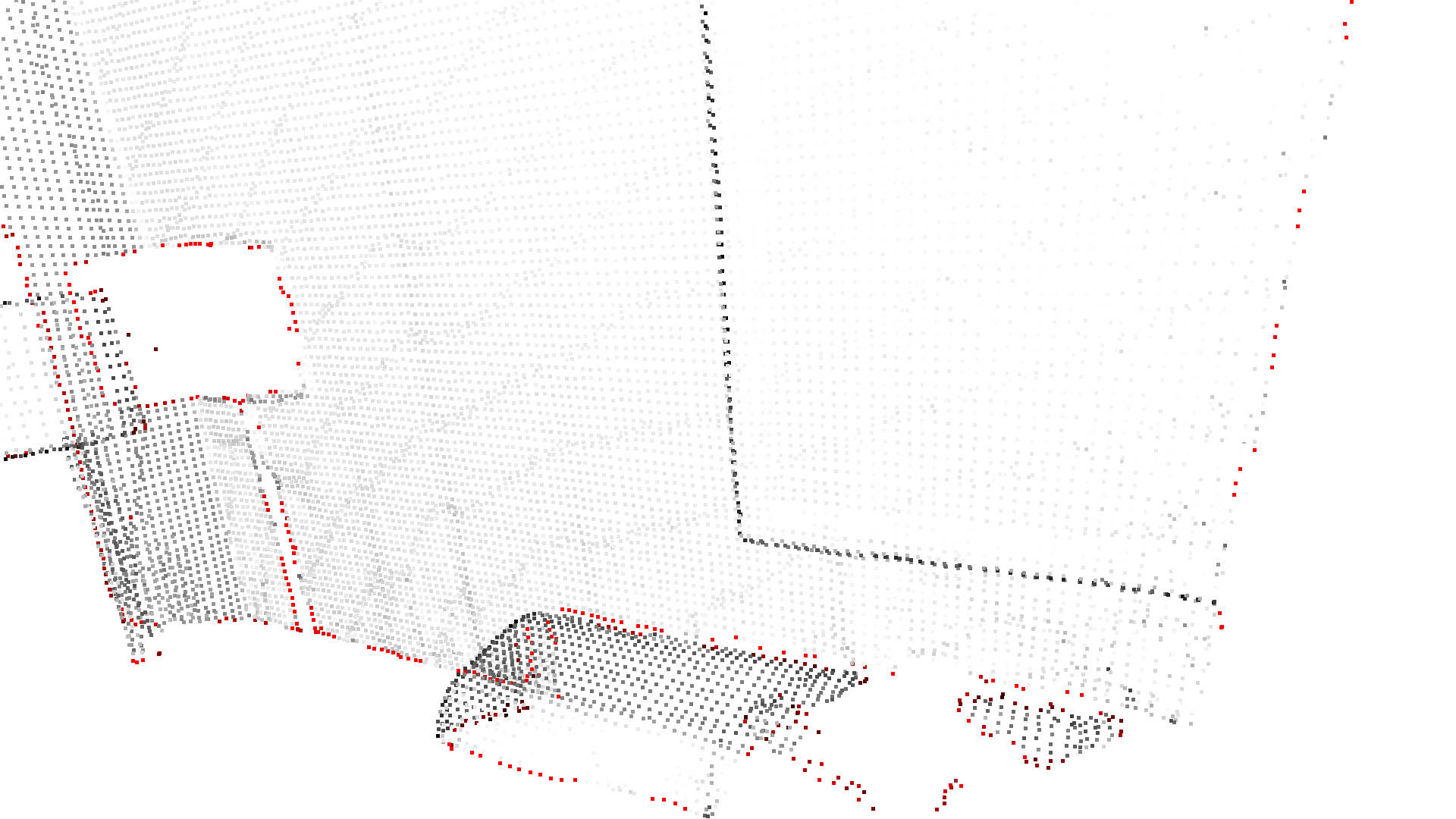# Point cloud outlier removal¶

When collecting data from scanning devices, the resulting point cloud tends to contain noise and artifacts that one would like to remove. This tutorial addresses the outlier removal features of Open3D.

## Prepare input data¶

A point cloud is loaded and downsampled using voxel_downsample.

:

print("Load a ply point cloud, print it, and render it")
sample_pcd_data = o3d.data.PCDPointCloud()
o3d.visualization.draw_geometries([pcd],
zoom=0.3412,
front=[0.4257, -0.2125, -0.8795],
lookat=[2.6172, 2.0475, 1.532],
up=[-0.0694, -0.9768, 0.2024])

print("Downsample the point cloud with a voxel of 0.02")
voxel_down_pcd = pcd.voxel_down_sample(voxel_size=0.02)
o3d.visualization.draw_geometries([voxel_down_pcd],
zoom=0.3412,
front=[0.4257, -0.2125, -0.8795],
lookat=[2.6172, 2.0475, 1.532],
up=[-0.0694, -0.9768, 0.2024])

Load a ply point cloud, print it, and render it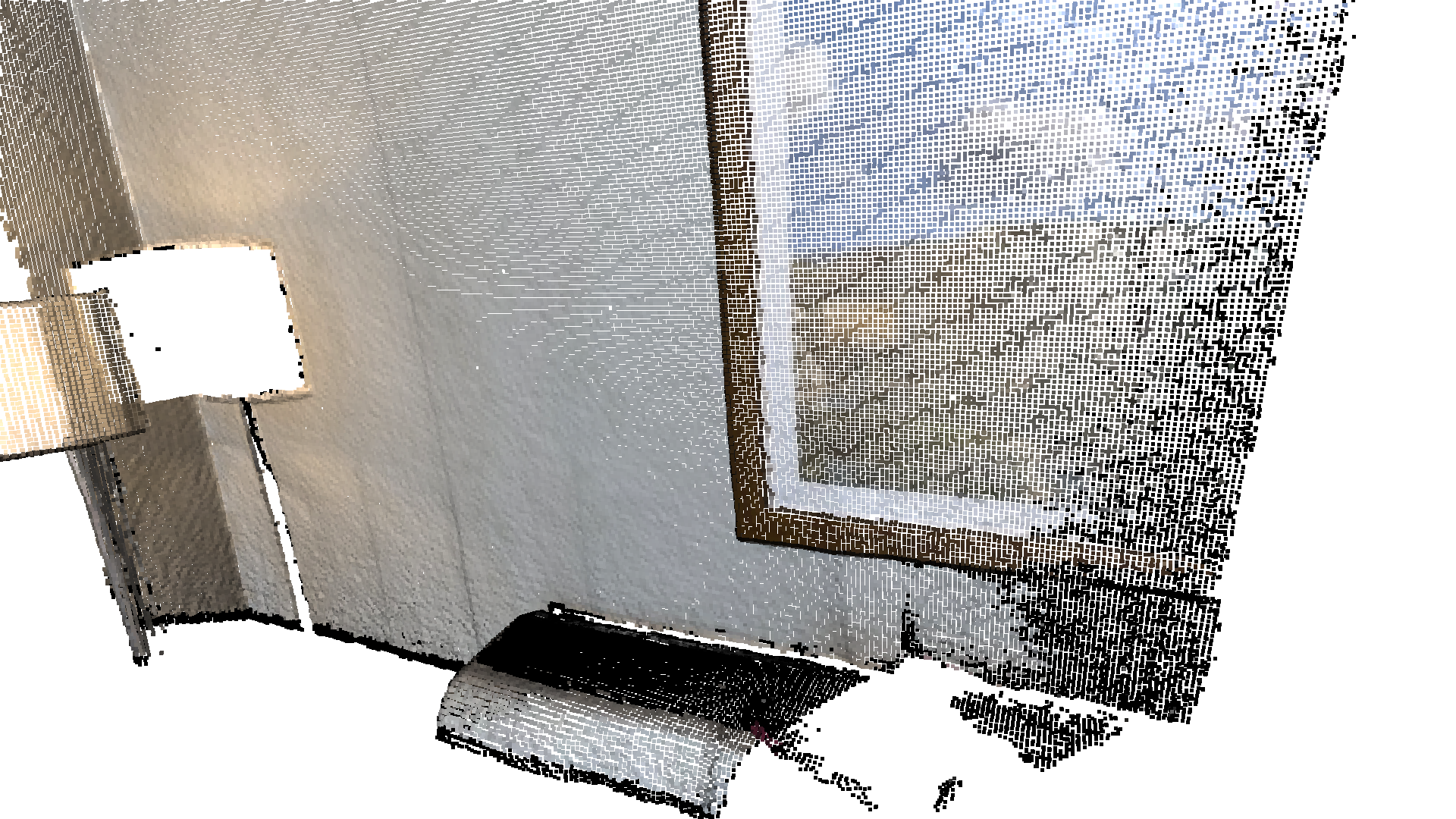Downsample the point cloud with a voxel of 0.02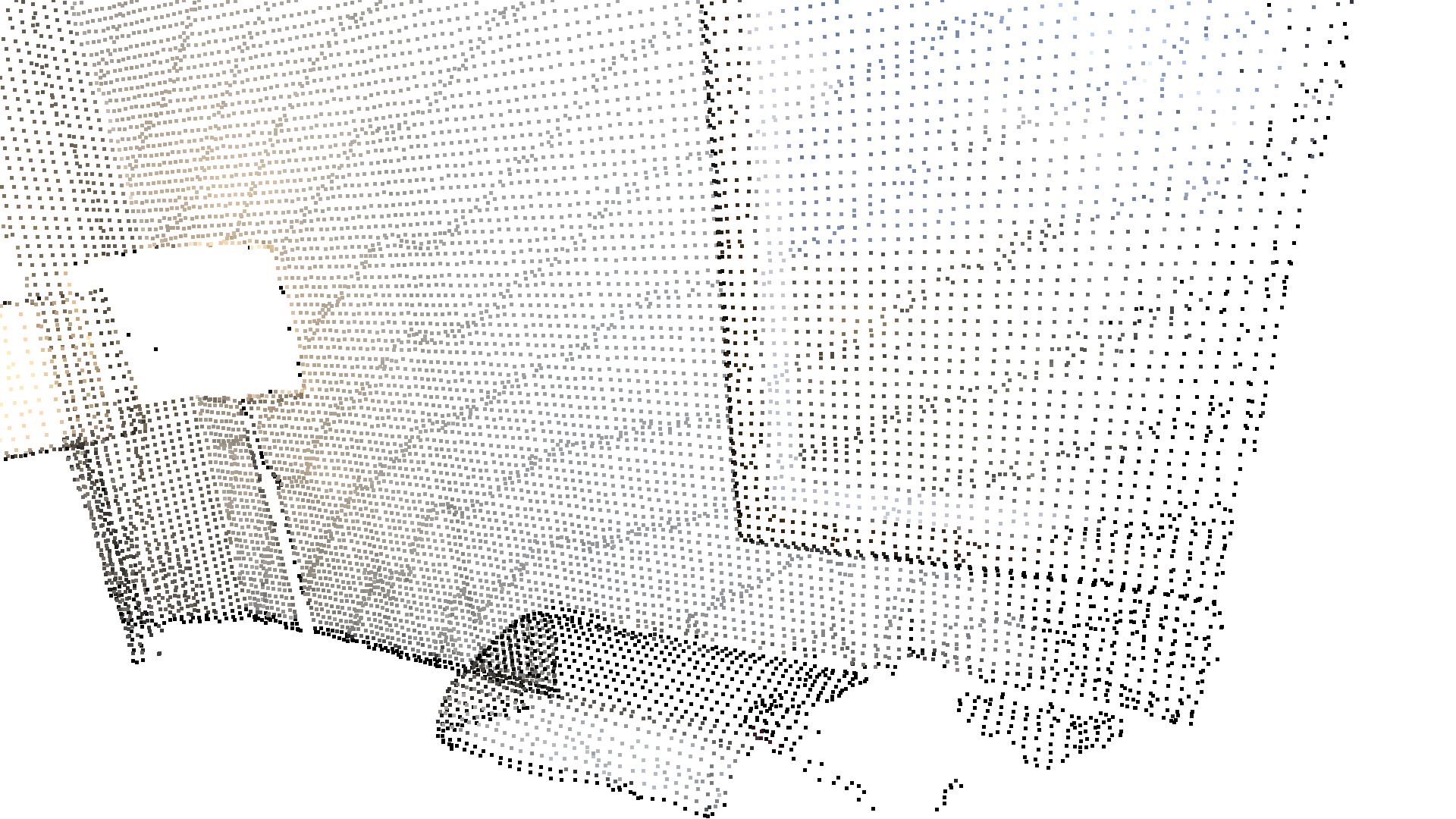Alternatively, use uniform_down_sample to downsample the point cloud by collecting every n-th points.

:

print("Every 5th points are selected")
uni_down_pcd = pcd.uniform_down_sample(every_k_points=5)
o3d.visualization.draw_geometries([uni_down_pcd],
zoom=0.3412,
front=[0.4257, -0.2125, -0.8795],
lookat=[2.6172, 2.0475, 1.532],
up=[-0.0694, -0.9768, 0.2024])

Every 5th points are selected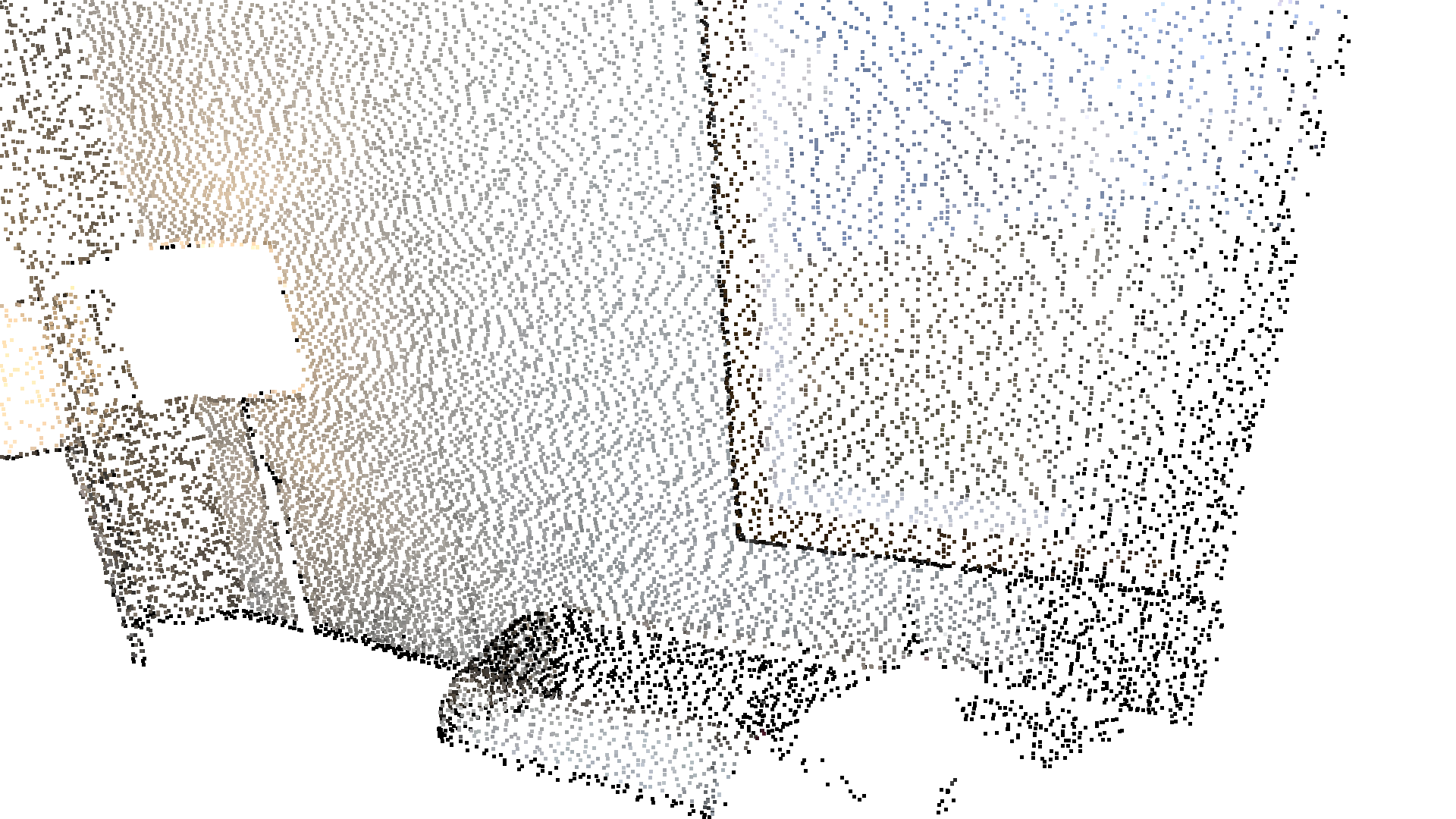## Select down sample¶

The following helper function uses select_by_index, which takes a binary mask to output only the selected points. The selected points and the non-selected points are visualized.

:

def display_inlier_outlier(cloud, ind):
inlier_cloud = cloud.select_by_index(ind)
outlier_cloud = cloud.select_by_index(ind, invert=True)

print("Showing outliers (red) and inliers (gray): ")
outlier_cloud.paint_uniform_color([1, 0, 0])
inlier_cloud.paint_uniform_color([0.8, 0.8, 0.8])
o3d.visualization.draw_geometries([inlier_cloud, outlier_cloud],
zoom=0.3412,
front=[0.4257, -0.2125, -0.8795],
lookat=[2.6172, 2.0475, 1.532],
up=[-0.0694, -0.9768, 0.2024])


## Statistical outlier removal¶

statistical_outlier_removal removes points that are further away from their neighbors compared to the average for the point cloud. It takes two input parameters:

• nb_neighbors, which specifies how many neighbors are taken into account in order to calculate the average distance for a given point.

• std_ratio, which allows setting the threshold level based on the standard deviation of the average distances across the point cloud. The lower this number the more aggressive the filter will be.

:

print("Statistical oulier removal")
cl, ind = voxel_down_pcd.remove_statistical_outlier(nb_neighbors=20,
std_ratio=2.0)
display_inlier_outlier(voxel_down_pcd, ind)

Statistical oulier removal
Showing outliers (red) and inliers (gray):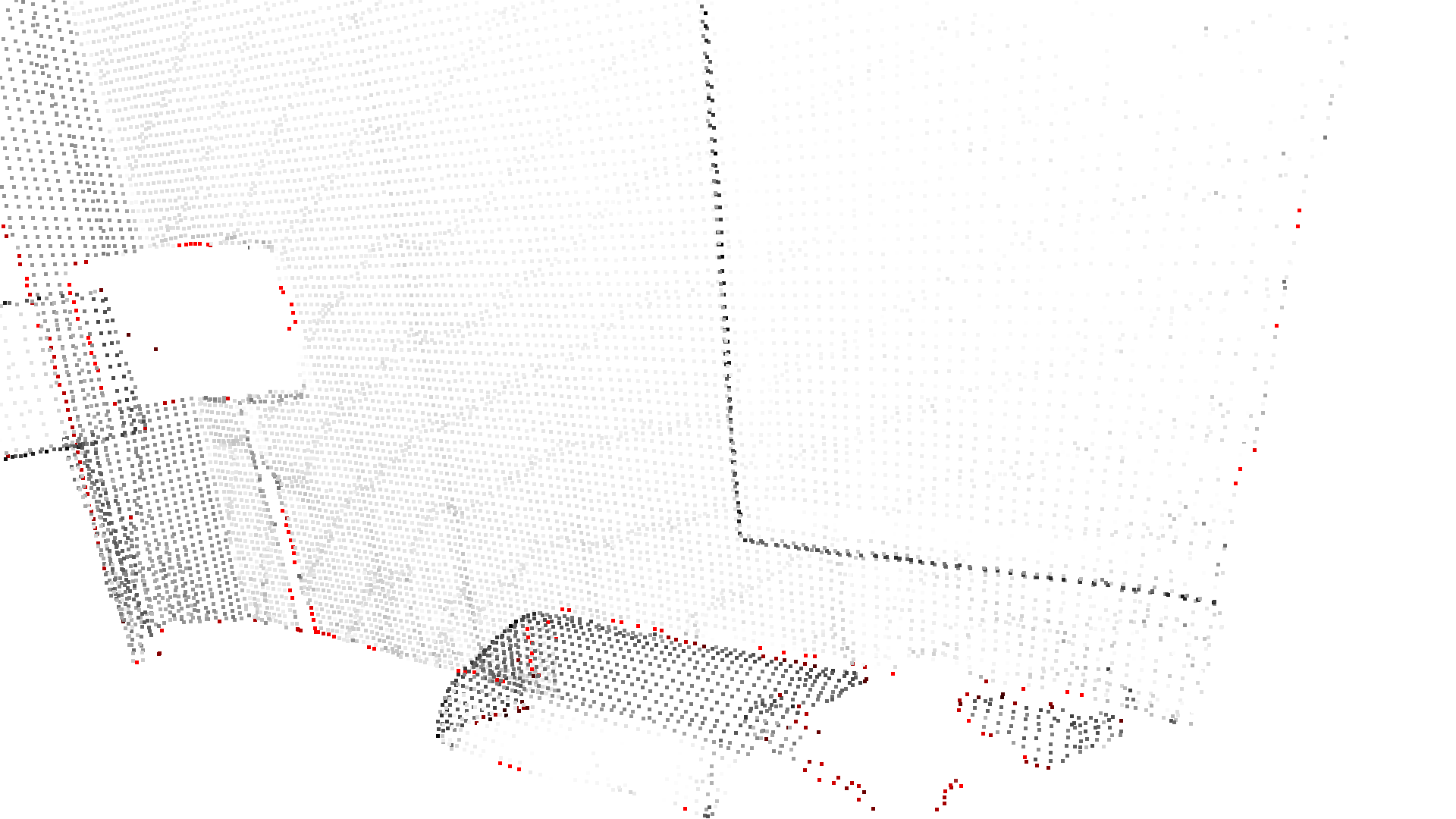radius_outlier_removal removes points that have few neighbors in a given sphere around them. Two parameters can be used to tune the filter to your data:

• nb_points, which lets you pick the minimum amount of points that the sphere should contain.

• radius, which defines the radius of the sphere that will be used for counting the neighbors.

:

print("Radius oulier removal")

Radius oulier removal